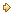Visitors Online: 77 | Sunday 20th October 2019

CBSE Guess > Papers > Question Papers > Class XII > 2004 > Economics > Delhi Set-III

ECONOMICS (Set III—Delhi)

Except for the following questions, all the remaining questions have been asked in Set I and Set II .

SECTION - A

Q. 1. Answer the following questions: 1X4
(i) Define variable costs.
(ii) What causes a movement along a supply curve of a good?
(iii) Why does the problem of choice arise?
(iv) Under which market form, a firm is a price-taker?

Q. 3. The price of a commodity is Rs. 12 per unit and its quantity supplied is 500 units. When its price rises to Rs. 15 per unit, its quantity supplied rises to 650 units. Calculate its price elasticity of supply. Is supply elastic? 3

Q. 6. What is meant by price elasticity of demand? Explain any two factors that affect it. 4

Q. 7. From the following table, calculate average variable cost of each given level of output: 4

 Output(units) 1 2 3 4 Marginal cost (Rs.) 70 60 62 72

SECTION - B

Q. 13. Answer the following questions: 1X4
(a) Give two examples of macro-economic studies.
(b) The balance of trade shows a deficit of Rs. 300 crores. The value of exports are Rs. 500 crores. What is the value of imports?
(c) Define fiscal deficit.
(d) Is the study of cotton textile industry a macro-economic study or a micro-economic study?

Q. 15. Calculate Gross National Disposable Income from the following data: 3

 Rs. (Crores) (i) Net factor income from abroad (ii) National income (iii) Net indirect taxes (iv) Net current transfers from rest of the world (v) Consumption of fixed capital (-) 10 1,000 80 150 100

Q. 16. In an economy, investment expenditure is increased by Rs. 700 crores. The marginal propensity to consume is 09. Calculate the total increase in income and consumption expenditures. 3

Q. 17. What is the relationship between average propensity to consume and average propensity to save? Can the value of average propensity to save be negative? If yes, when? 3

Q. 22. From the following data, calculate Gross National Product At Market Price by (i) income method and (ii) expenditure method. 3, 3

 Rs. (Crores) (i) Net domestic capital formation (ii) Compensation of employees (ii) Net indirect taxes (iv) Profits (v) Rent (vi) Private final consumption expenditure (vii) Consumption of fixed capital (viii) Government final consumption expenditure (ix) Interest (x) Mixed income of self employed (xi) Net factor income from abroad (xii) Net exports 375 600 150 450 200 1,100 115 700 250 500 (-) 15 (-) 25

 Economics 2004 Question Papers Class XII Delhi Outside Delhi Compartment Delhi Compartment Outside DelhiSet ISet ISet ISet ISet IISet IISet IISet IISet IIISet III

CBSE 2004 Question Papers Class XII